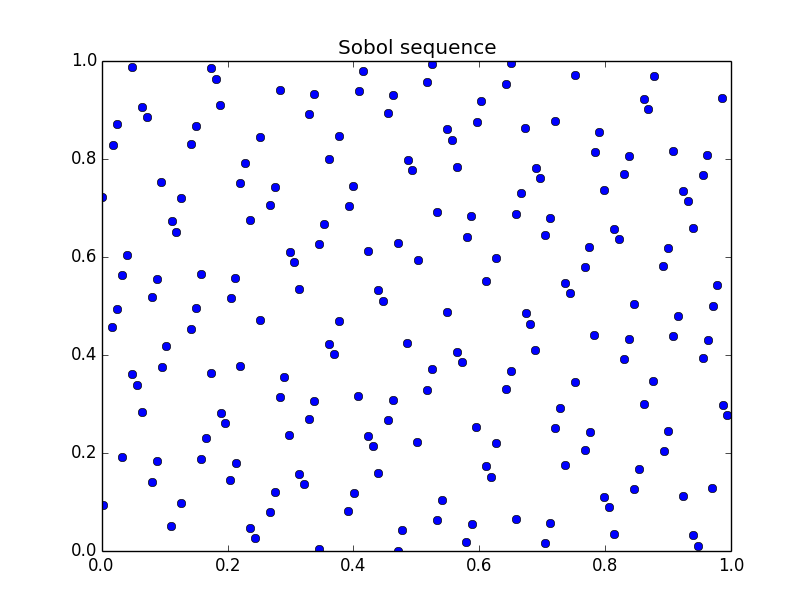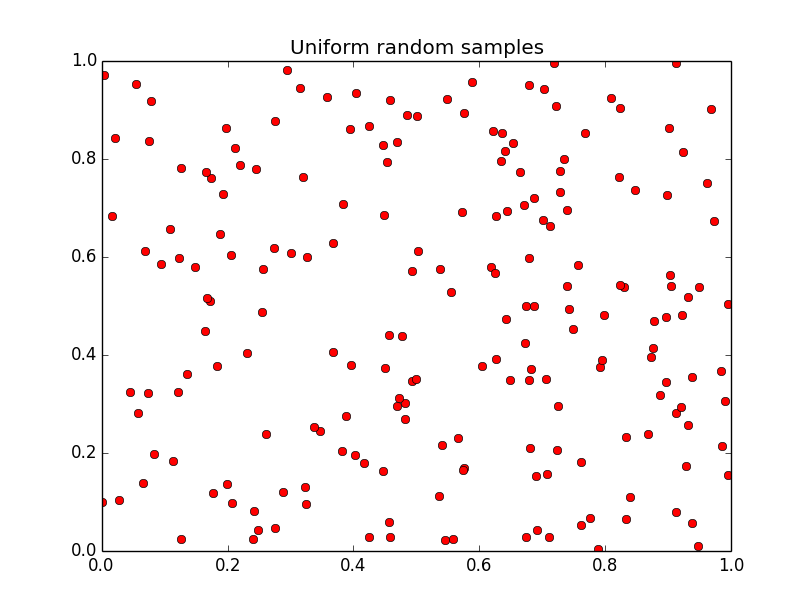# Sobol sequences¶

A Sobol sequence is a low discrepancy quasi-random sequence. Sobol sequences were designed to cover the unit hypercube with lower discrepancy than completely random sampling (e.g. Random Search). Optunity supports Sobol sequences in up to 40 dimensions (e.g. 40 hyperparameters).

The figures below show the differences between a Sobol sequence and sampling uniformly at random. These figures can be recreated using the code in bin/examples/python/sobol_vs_random.py.200 points sampled in 2D with a Sobol sequence.200 points sampled in 2D uniformly at random.

The mathematical details on Sobol sequences are available in the following papers: [SOBOL], [SOBOL2], [ANTONOV], [BRATLEY], [FOX].

 [SOBOL] Ilya Sobol, USSR Computational Mathematics and Mathematical Physics, Volume 16, pages 236-242, 1977.
 [SOBOL2] Ilya Sobol, Levitan, The Production of Points Uniformly Distributed in a Multidimensional Cube (in Russian), Preprint IPM Akad. Nauk SSSR, Number 40, Moscow 1976.
 [ANTONOV] Antonov, Saleev, USSR Computational Mathematics and Mathematical Physics, Volume 19, 1980, pages 252 - 256.
 [BRATLEY] Paul Bratley, Bennett Fox, Algorithm 659: Implementing Sobol’s Quasirandom Sequence Generator, ACM Transactions on Mathematical Software, Volume 14, Number 1, pages 88-100, 1988.
 [FOX] Bennett Fox, Algorithm 647: Implementation and Relative Efficiency of Quasirandom Sequence Generators, ACM Transactions on Mathematical Software, Volume 12, Number 4, pages 362-376, 1986.